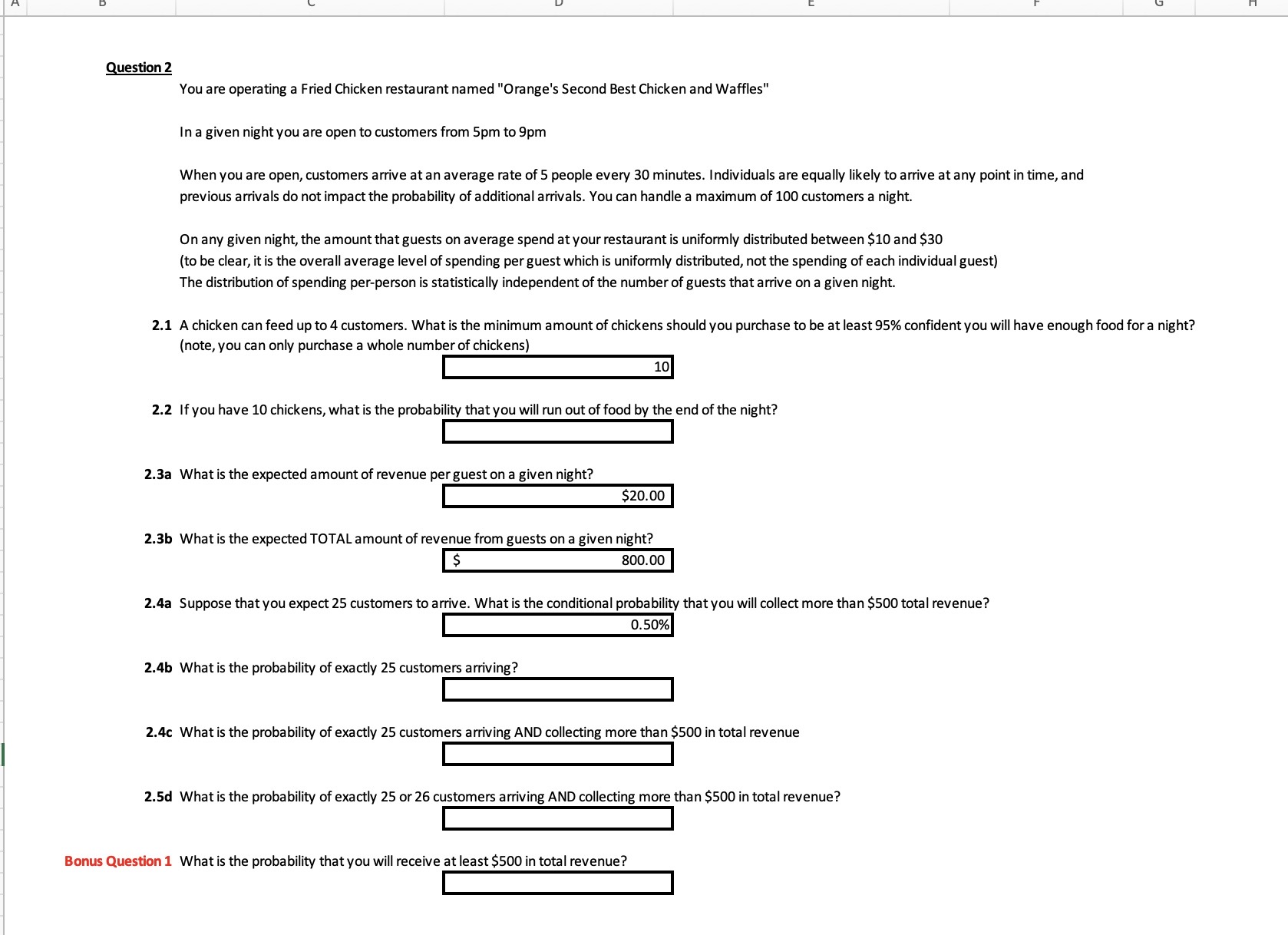Home / Expert Answers / Statistics and Probability / you-are-operating-a-fried-chicken-restaurant-named-34-orange-39-s-second-best-chicken-and-waffles-34-pa966

# (Solved): You are operating a Fried Chicken restaurant named "Orange's Second Best Chicken and Waffles" In a ...You are operating a Fried Chicken restaurant named "Orange's Second Best Chicken and Waffles" In a given night you are open to customers from to When you are open, customers arrive at an average rate of 5 people every 30 minutes. Individuals are equally likely to arrive at any point in time, and previous arrivals do not impact the probability of additional arrivals. You can handle a maximum of 100 customers a night. On any given night, the amount that guests on average spend at your restaurant is uniformly distributed between and (to be clear, it is the overall average level of spending per guest which is uniformly distributed, not the spending of each individual guest) The distribution of spending per-person is statistically independent of the number of guests that arrive on a given night. (note, you can only purchase a whole number of chickens) 2.2 If you have 10 chickens, what is the probability that you will run out of food by the end of the night? 2.3a What is the expected amount of revenue per guest on a given night? 2.3b What is the expected TOTAL amount of revenue from guests on a given night? 2.4a Suppose that you expect 25 customers to arrive. What is the conditional probability that you will collect more than total revenue? 2.4b What is the probability of exactly 25 customers arriving? 2.4c What is the probability of exactly 25 customers arriving AND collecting more than in total revenue 2.5d What is the probability of exactly 25 or 26 customers arriving AND collecting more than in total revenue? Bonus Question 1 What is the probability that you will receive at least in total revenue?

We have an Answer from Expert

2.1 To determine the minimum amount of chickens you should purchase to be at least 95% confident you will have enough food for the night, we need to calculate the expected number of customers that will arrive in the given four-hour period, and then use that information to calculate the number of chickens needed.

The expected number of customers that will arrive during a four-hour period is:
Expected number of customers = (5 customers/30 minutes) x (2 x 4 hours) = 40 customers

Since a chicken can feed up to 4 customers, we need to ensure that we have at least   chickens.

To be 95% confident that we have enough food, we need to calculate the number of chickens that will be sufficient with 95% probability. We can use the normal distribution and the following formula to determine this:

Number of chickens = Mean + Z-score x Standard deviation

The mean is 10 chickens, and the standard deviation can be calculated as follows:

Standard deviation

where sqrt(40) is the square root of the expected number of customers.

The Z-score corresponding to a 95% confidence level is 1.96.

Thus, the number of chickens needed is:

Number of chickens

Since we can only purchase a whole number of chickens, we need to round up to the nearest whole number and purchase 14 chickens to be 95% confident we will have enough food for the night.

2.2 If we have 10 chickens, the maximum number of customers we can serve is 10 x 4 = 40 customers.

The expected number of customers in a given night is 5 customers/30 minutes x 8 30-minute intervals = 40 customers, which is the same as the maximum number of customers we can serve with the available chickens.

However, the actual number of customers that arrive in a given night can vary. We can use the Poisson distribution to calculate the probability that more than 40 customers will arrive in the given night.

The probability of more than 40 customers arriving can be calculated as follows:

where X is the Poisson distribution with a mean of 40 customers.

Using a Poisson calculator, we find that    so

Therefore, the probability of running out of food with 10 chickens is approximately 5.5%.
We have an Answer from Expert